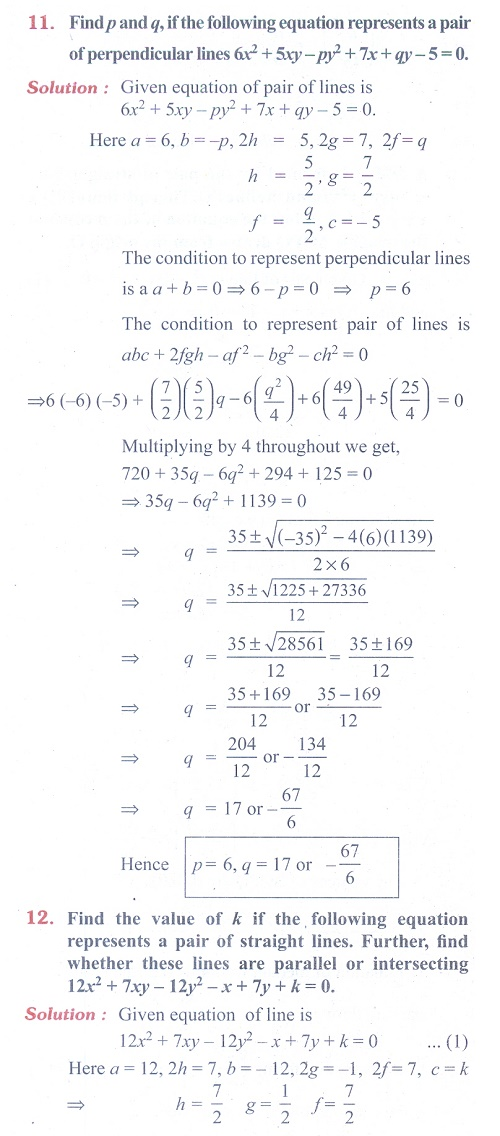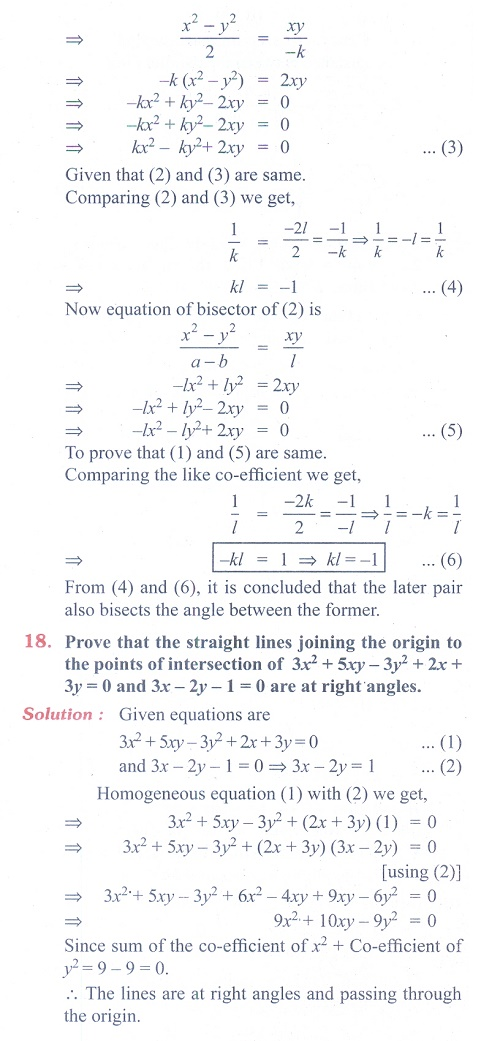Home | | Maths 11th std | Exercise 6.4: Pair of Straight Lines

# Exercise 6.4: Pair of Straight Lines

Maths Book back answers and solution for Exercise questions - Mathematics : Two Dimensional Analytical Geometry: Pair of Straight LinesTags : Problem Questions with Answer, Solution | Mathematics , 11th Mathematics : UNIT 6 : Two Dimensional Analytical Geometry
Study Material, Lecturing Notes, Assignment, Reference, Wiki description explanation, brief detail
11th Mathematics : UNIT 6 : Two Dimensional Analytical Geometry : Exercise 6.4: Pair of Straight Lines | Problem Questions with Answer, Solution | Mathematics